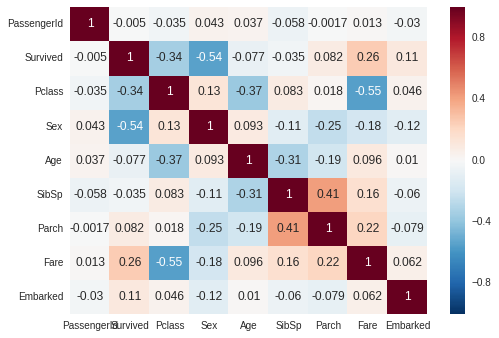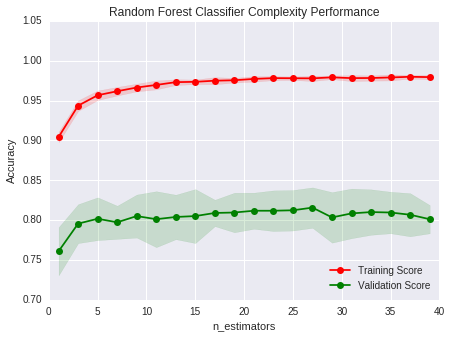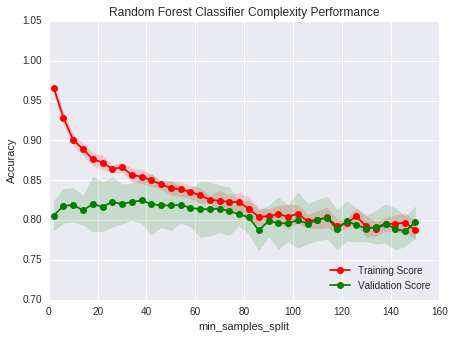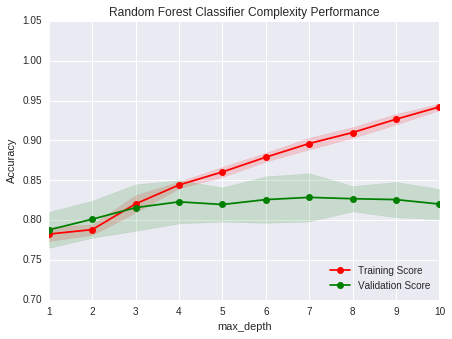Titanic_writeup

# Kaggle Titanic Survival Prediction¶

This is from the Kaggle tutorial competition, Titanic: Machine Learning from Disaster. A lot of the codes are adapted from Dataquest lesson and Udacity Machine Learning Engineer Nanodegree.

In this example, we'll predict survival of passengers by looking at different passenger data, such as age, gender, etc. We'll try three different models---logistic regression, random forest, and extreme gradient boosting.

## Importing necessary packages¶

We'll need pandas and numpy to work with data frames and do math manipulations. We'll use sklearn for machine learning models. Matplotlib will be also useful in case we want to plot anything!

In :
import pandas as pd
import numpy as np
from sklearn.linear_model import LogisticRegression
from sklearn.ensemble import RandomForestClassifier
from sklearn.model_selection import cross_val_score, KFold
import matplotlib.pyplot as plt
%matplotlib inline


## Data import and munging¶

Now, time to import data and turn all columns into numeric data.

#### Import data¶

Import train and test data sets from local csv files, then look at first few rows of each.

In :
train = pd.read_csv('train.csv', header=0)

Out:
PassengerId Survived Pclass Name Sex Age SibSp Parch Ticket Fare Cabin Embarked
0 1 0 3 Braund, Mr. Owen Harris male 22.0 1 0 A/5 21171 7.2500 NaN S
1 2 1 1 Cumings, Mrs. John Bradley (Florence Briggs Th... female 38.0 1 0 PC 17599 71.2833 C85 C
2 3 1 3 Heikkinen, Miss. Laina female 26.0 0 0 STON/O2. 3101282 7.9250 NaN S
3 4 1 1 Futrelle, Mrs. Jacques Heath (Lily May Peel) female 35.0 1 0 113803 53.1000 C123 S
4 5 0 3 Allen, Mr. William Henry male 35.0 0 0 373450 8.0500 NaN S
In :
test = pd.read_csv('test.csv', header=0)

Out:
PassengerId Pclass Name Sex Age SibSp Parch Ticket Fare Cabin Embarked
0 892 3 Kelly, Mr. James male 34.5 0 0 330911 7.8292 NaN Q
1 893 3 Wilkes, Mrs. James (Ellen Needs) female 47.0 1 0 363272 7.0000 NaN S
2 894 2 Myles, Mr. Thomas Francis male 62.0 0 0 240276 9.6875 NaN Q
3 895 3 Wirz, Mr. Albert male 27.0 0 0 315154 8.6625 NaN S
4 896 3 Hirvonen, Mrs. Alexander (Helga E Lindqvist) female 22.0 1 1 3101298 12.2875 NaN S

#### Numeric data type¶

Let's turn sex and embarked into numeric data. Also, we won't bother with Name, Ticket, and Cabin right now, so let's remove those columns.
'female' = 0, 'male' = 1
'S' = 0, 'C' = 1, 'Q' = 2

In :
train = train.drop(['Name','Ticket','Cabin'],1)
test = test.drop(['Name','Ticket','Cabin'],1)

train.Sex = train.Sex.map({'female':0, 'male':1}).astype(int)
test.Sex = test.Sex.map({'female':0, 'male':1}).astype(int)

train.Embarked = train.Embarked.fillna('S')
test.Embarked = test.Embarked.fillna('S')
train.Embarked = train.Embarked.map({'S':0, 'C':1, 'Q':2})
test.Embarked = test.Embarked.map({'S':0, 'C':1, 'Q':2})

In :
train.head()

Out:
PassengerId Survived Pclass Sex Age SibSp Parch Fare Embarked
0 1 0 3 1 22.0 1 0 7.2500 0
1 2 1 1 0 38.0 1 0 71.2833 1
2 3 1 3 0 26.0 0 0 7.9250 0
3 4 1 1 0 35.0 1 0 53.1000 0
4 5 0 3 1 35.0 0 0 8.0500 0
In :
test.head()

Out:
PassengerId Pclass Sex Age SibSp Parch Fare Embarked
0 892 3 1 34.5 0 0 7.8292 2
1 893 3 0 47.0 1 0 7.0000 0
2 894 2 1 62.0 0 0 9.6875 2
3 895 3 1 27.0 0 0 8.6625 0
4 896 3 0 22.0 1 1 12.2875 0

#### Quick break: correlation¶

Now all our data is numeric. A useful concept to look at is correlation. It informs us of which features are good predictors. We'll look at it both as a table and a plot. We'll have to import another package to make this plot.

In :
import seaborn as sb

In :
corr = train.corr()
sb.heatmap(corr, annot=True)
plt.show()We can see the sex and passenger class and fare are three biggest predictors. (well, fare and passenger class are most likely related, but whatever...).

#### Dealing with missing values¶

In :
print(train.isnull().sum())
print(test.isnull().sum())

PassengerId      0
Survived         0
Pclass           0
Sex              0
Age            177
SibSp            0
Parch            0
Fare             0
Embarked         0
dtype: int64
PassengerId     0
Pclass          0
Sex             0
Age            86
SibSp           0
Parch           0
Fare            1
Embarked        0
dtype: int64


Earlier, we filled NA values for Embarked column. I didn't do anything fancy. I already knew S was most common port, and I just filled the missing values with that.
As shown above, we still have a lot of missing values for Age. We can try to do something more fancy with age, since it appears age is significantly correlated with few other features, namely Pclass and SibSp.
There's one missing fare value in test data set. Let's just fill this in with median of same Pclass.

In :
for i in range(len(train.Age)):
if np.isnan(train.Age[i]):
if train.SibSp[i] == 0:
train.loc[i,"Age"] = np.nanmedian(train.Age[(train.Pclass==train.Pclass[i]) & (train.SibSp == 0)])
elif train.SibSp[i] > 0:
train.loc[i,"Age"] = np.nanmedian(train.Age[(train.Pclass==train.Pclass[i]) & (train.SibSp > 0)])
else:
train.loc[i,"Age"] = np.nanmedian(train.Age[train.Pclass==train.Pclass[i]])
for i in range(len(test.Age)):
if np.isnan(test.Age[i]):
if test.SibSp[i] == 0:
test.loc[i,"Age"] = np.nanmedian(train.Age[(train.Pclass == test.Pclass[i]) & (train.SibSp == 0)])
elif test.SibSp[i] > 0:
test.loc[i,"Age"] = np.nanmedian(train.Age[(train.Pclass == test.Pclass[i]) & (train.SibSp > 0)])
else:
test.loc[i,"Age"] = np.nanmedian(train.Age[train.Pclass == test.Pclass[i]])

test.loc[test.Fare.isnull(),"Fare"] = np.median(train.Fare[train.Pclass == test.Pclass[test.Fare.isnull()].iloc])


We can do more, such as extracting out titles from names (Mr., Mrs., Dr., etc.) or calculating family size (SibSp + Parch). Feature engineering is an important portion of machine learning, but I haven't found much helpful feature for this example. If you're interested in how to implement them anyway, see Dataquest mission.

## Machine Learning¶

Now that we have the data ready, it's time to train our data. We most likely don't want to use all features as predictors as it would overfit. We can play with different combinations of predictors, but let's just with try the below combination.

In :
features = ["Pclass", "Sex", "Fare", "Age"]


Let's create a function that takes an algorithm, trains on a data with features X and labels Y, and gives us the accuracy on 5-fold cross validation set.

In :
def cv_accuracy(X, Y, algorithm):
kf = KFold(n_splits=5, random_state=1, shuffle=True)
kf.get_n_splits(train)

idx_shuf = []
predictions = []
for tr, cv in kf.split(X):
idx_shuf.append(cv)
x_train = X.iloc[tr,:]
y_train = Y.iloc[tr]
algorithm.fit(x_train, y_train)
cv_pred = algorithm.predict(X.iloc[cv,:])
predictions.append(cv_pred)
idx_shuf = np.concatenate(idx_shuf, axis=0)
predictions = np.concatenate(predictions, axis=0)
accuracy = np.array(np.round(predictions) == Y.iloc[idx_shuf]).mean()

return accuracy


#### Logistic regression¶

Let's first look at logistic regression (see Wikipedia page for information on it).

In :
alg = LogisticRegression(random_state=2015)

In :
accuracy = cv_accuracy(train[features], train['Survived'], alg)
print(accuracy) #0.7845117845

0.79012345679


Accuracy of 79.0% is okay, but we can try to improve it. Let's try this on test set and see what we get.

In :
predictions = alg.predict(test[features])
submission = pd.DataFrame({
"PassengerId": test['PassengerId'],
"Survived": predictions})
submission.to_csv("prediction_logistic.csv", index=False)


This gets 75.1% accuracy on the leaderboard, which's pretty close to the cross validation accuracy.

#### Random Forest¶

Let's try a slightly more complicated model called Random Forest Ensemble using the same predictors.

In :
alg = RandomForestClassifier(random_state=13, n_estimators=15, min_samples_split=5)

In :
accuracy = cv_accuracy(train[features], train['Survived'], alg)
print(accuracy)

0.812570145903


We get 81.3%, a pretty good improvement over the simple logistic regression. Now, let's apply this to our test set and see what we get.

In :
predictions = alg.predict(test[features])
submission = pd.DataFrame({
"PassengerId": test['PassengerId'],
"Survived": predictions})
submission.to_csv("prediction_randomforest.csv", index=False)


This actually gives 74.6% accuracy, lower than our logistic regression! Maybe it's overfitting. Let's see if we can play with parameters to control our overfitting.

#### Parameter Tuning¶

I wrote some codes adapted from Udacity's Maching Learning Engineer Nanodegree to visualize change in accuracy in terms of change in parameter complexity. I'll look at three parameters in specific: n_estimators, min_samples_split, and max_depth.

In :
import parameter_optimization as po

In :
po.NEstimatorComplexity(train[features],train['Survived'],'n_estimators')
po.NEstimatorComplexity(train[features],train['Survived'],'min_samples_split')
po.NEstimatorComplexity(train[features],train['Survived'],'max_depth')We can see that n_estimator and min_sample_split give relatively consistent cross-validation accuracy within some range. max_depth has a minor underfitting at very low values and overfitting at high values.
It's pretty hard to judge from these plots which parameter values are the best. Let's try something called a grid search, which is a method of looking at the exhaustive combinations of different parameter values to find the optimum parameters for the cross-validation accuracy. (Let's also add min_samples_leaf in there too.)
For questions on what all these parameters mean, refer to sklearn doc.

In :
from sklearn.metrics import make_scorer
from sklearn.model_selection import GridSearchCV
acc_score = make_scorer(po.acc_score_func)

In :
from sklearn.model_selection import ShuffleSplit
clf = RandomForestClassifier()
params = {'n_estimators': range(5,16),'min_samples_split': range(10,51,1),
'max_depth': range(1,11), 'min_samples_leaf': range(1,11)}
cv = ShuffleSplit(n_splits = 10, test_size = 0.2, random_state = 2017)
grid = GridSearchCV(clf, params, scoring=acc_score, cv=cv.get_n_splits(train))
grid = grid.fit(train[features],train['Survived'])

In :
grid.best_estimator_.get_params()

Out:
{'bootstrap': True,
'class_weight': None,
'criterion': 'gini',
'max_depth': 9,
'max_features': 'auto',
'max_leaf_nodes': None,
'min_impurity_split': 1e-07,
'min_samples_leaf': 1,
'min_samples_split': 18,
'min_weight_fraction_leaf': 0.0,
'n_estimators': 15,
'n_jobs': 1,
'oob_score': False,
'random_state': None,
'verbose': 0,
'warm_start': False}

It has found the following optimum parameters:
max_depth = 7, n_estimators = 15, min_samples_split = 14, min_samples_leaf = 1
Let's run the algorithm again using those parameter values!

In :
alg = RandomForestClassifier(random_state=13, max_depth=8, n_estimators=12, min_samples_split=14, min_samples_leaf=3)

In :
accuracy = cv_accuracy(train[features], train['Survived'], alg)
print(accuracy)

0.829405162738


82.9%, which is a small increase from our non-optimized classifier. That's a good sign!

In :
predictions = alg.predict(test[features])
submission = pd.DataFrame({
"PassengerId": test['PassengerId'],
"Survived": predictions})
submission.to_csv("prediction_randomforest_optimized.csv", index=False)


This submission gave a accuracy of 77.0, which is a 2.4% increase from our last one, just by tuning the parameters. Good job!

Now let's try a technique called gradient boosting using the library XGBoost. XGBoost doc has a good introduction to gradient boosting as well.

In :
import xgboost as xgb

/home/mbllinux/anaconda2/envs/tf3/lib/python3.5/site-packages/sklearn/cross_validation.py:44: DeprecationWarning: This module was deprecated in version 0.18 in favor of the model_selection module into which all the refactored classes and functions are moved. Also note that the interface of the new CV iterators are different from that of this module. This module will be removed in 0.20.
"This module will be removed in 0.20.", DeprecationWarning)

In :
param = {'max_depth':4, 'gamma':0.5, 'min_child_weight':1,
'eta':1, 'silent':0, 'objective':'binary:logistic',
'silent':1, 'seed':29}

In :
from sklearn.model_selection import ShuffleSplit


Let's re-write our accuracy function for XGBoost. This should look basically the same as our other one.

In :
def xgb_cv_accuracy(X, Y, param):
num_round = 3
kf = KFold(n_splits=5, random_state=1, shuffle=True)
kf.get_n_splits(train)

idx_shuf = []
predictions = []
for tr, cv in kf.split(X):
idx_shuf.append(cv)

dtrain = xgb.DMatrix(X.iloc[tr],label=Y.iloc[tr])
dtest = xgb.DMatrix(X.iloc[cv],label=Y.iloc[cv])

watchlist  = [(dtest,'validation'),(dtrain,'train')]
bst = xgb.train(param, dtrain, num_round, watchlist)

cv_pred = bst.predict(dtest)
predictions.append(cv_pred)
idx_shuf = np.concatenate(idx_shuf, axis=0)
predictions = np.concatenate(predictions, axis=0)
accuracy = np.array(np.round(predictions) == Y.iloc[idx_shuf]).mean()

return accuracy

In :
accuracy = xgb_cv_accuracy(train[features], train['Survived'],param)
print(accuracy)

	validation-error:0.21229	train-error:0.147472
	validation-error:0.223464	train-error:0.146067
	validation-error:0.217877	train-error:0.140449
	validation-error:0.219101	train-error:0.154278
	validation-error:0.207865	train-error:0.140252
	validation-error:0.219101	train-error:0.134642
	validation-error:0.168539	train-error:0.168303
	validation-error:0.219101	train-error:0.158485
	validation-error:0.196629	train-error:0.148668
	validation-error:0.157303	train-error:0.162693
	validation-error:0.162921	train-error:0.152875
	validation-error:0.157303	train-error:0.143058
	validation-error:0.196629	train-error:0.173913
	validation-error:0.162921	train-error:0.175316
	validation-error:0.179775	train-error:0.158485
0.805836139169


Without tuning any parameter, we got 80.6% cross-validation accuracy. It seems lower than the random forest ensemble, but let's look at the test accuracy.

In :
dtrain = xgb.DMatrix(train[features],label=train['Survived'])
bst = xgb.train(param, dtrain)
predictions = np.round(bst.predict(xgb.DMatrix(test[features]))).astype(int)
submission = pd.DataFrame({
"PassengerId": test['PassengerId'],
"Survived": predictions})
submission.to_csv("prediction_xgboost.csv", index=False)


We get 76.6% test accuracy, close to our last result. Not bad!

Next step should be clear, let's do grid search on xgboost! Unfortunately, xgboost library doesn't come with a grid search technique. However, someone from Kaggle graciously provided an easy way to make xgboost compatible with sklearn classifiers.
Let's do grid search with bunch of parameters... why not if you have the time, right?

In :
import XGBoost_Classfier as xgb_clf

In :
clf = xgb_clf.XGBoostClassifier(num_class=2, silent=1, seed=29)
params = {'num_boost_round': [50,100,150,200],'max_depth': range(4,11), 'eta': np.logspace(-2,-0.4,num=9,base=10.),
'gamma': np.logspace(-1,1,num=11,base=10.), 'min_child_weight': range(1,6)}
cv = ShuffleSplit(n_splits = 10, test_size = 0.2, random_state = 2017)
clf = GridSearchCV(clf, params, cv=cv.get_n_splits(train))
clf.fit(train[features],train['Survived'])

Out:
GridSearchCV(cv=10, error_score='raise',
estimator=<XGBoost_Classfier.XGBoostClassifier object at 0x7f7665116208>,
fit_params={}, iid=True, n_jobs=1,
param_grid={'gamma': array([  0.1    ,   0.15849,   0.25119,   0.39811,   0.63096,   1.     ,
1.58489,   2.51189,   3.98107,   6.30957,  10.     ]), 'num_boost_round': [50, 100, 150, 200], 'eta': array([ 0.01   ,  0.01585,  0.02512,  0.03981,  0.0631 ,  0.1    ,
0.15849,  0.25119,  0.39811]), 'max_depth': range(4, 11), 'min_child_weight': range(1, 6)},
pre_dispatch='2*n_jobs', refit=True, return_train_score=True,
scoring=None, verbose=0)
In :
best_parameters, score, _ = max(clf.grid_scores_, key=lambda x: x)
print(best_parameters)

/home/mbllinux/anaconda2/envs/tf3/lib/python3.5/site-packages/sklearn/model_selection/_search.py:667: DeprecationWarning: The grid_scores_ attribute was deprecated in version 0.18 in favor of the more elaborate cv_results_ attribute. The grid_scores_ attribute will not be available from 0.20
DeprecationWarning)

{'gamma': 0.39810717055349731, 'max_depth': 4, 'eta': 0.15848931924611143, 'num_boost_round': 50, 'min_child_weight': 2}


Again, we use the parameters to see what we get on the test set!

In :
dtrain = xgb.DMatrix(train[features],label=train['Survived'])
bst = xgb.train(best_parameters, dtrain)
predictions = np.round(bst.predict(xgb.DMatrix(test[features]))).astype(int)
submission = pd.DataFrame({
"PassengerId": test['PassengerId'],
"Survived": predictions})
submission.to_csv("prediction_xgboost_opt.csv", index=False)


This gives 78.5% test accuracy, nice!

I see that on the Kaggle Public Leaderboard, the benchmark for Gender, Price and Class Based Model is pretty high (78.0%)! Are we overfitting by using the age feature? Well... let's try it without it!

In :
features = ["Pclass", "Sex", "Fare"]
params = {'num_boost_round': [50,100,150,200],'max_depth': range(4,11), 'eta': np.logspace(-2,-0.4,num=9,base=10.),
'gamma': np.logspace(-1,1,num=11,base=10.), 'min_child_weight': range(1,6)}
clf = xgb_clf.XGBoostClassifier(num_class=2, silent=1, seed=29)
cv = ShuffleSplit(n_splits = 10, test_size = 0.2, random_state = 2017)
clf = GridSearchCV(clf, params, cv=cv.get_n_splits(train))
clf.fit(train[features],train['Survived'])

Out:
GridSearchCV(cv=10, error_score='raise',
estimator=<XGBoost_Classfier.XGBoostClassifier object at 0x7f76669e1cf8>,
fit_params={}, iid=True, n_jobs=1,
param_grid={'gamma': array([  0.1    ,   0.15849,   0.25119,   0.39811,   0.63096,   1.     ,
1.58489,   2.51189,   3.98107,   6.30957,  10.     ]), 'num_boost_round': [50, 100, 150, 200], 'eta': array([ 0.01   ,  0.01585,  0.02512,  0.03981,  0.0631 ,  0.1    ,
0.15849,  0.25119,  0.39811]), 'max_depth': range(4, 11), 'min_child_weight': range(1, 6)},
pre_dispatch='2*n_jobs', refit=True, return_train_score=True,
scoring=None, verbose=0)
In :
best_parameters, score, _ = max(clf.grid_scores_, key=lambda x: x)
print(best_parameters)

/home/mbllinux/anaconda2/envs/tf3/lib/python3.5/site-packages/sklearn/model_selection/_search.py:667: DeprecationWarning: The grid_scores_ attribute was deprecated in version 0.18 in favor of the more elaborate cv_results_ attribute. The grid_scores_ attribute will not be available from 0.20
DeprecationWarning)

{'gamma': 0.25118864315095801, 'max_depth': 5, 'eta': 0.3981071705534972, 'num_boost_round': 200, 'min_child_weight': 1}

In :
dtrain = xgb.DMatrix(train[features],label=train['Survived'])
bst = xgb.train(best_parameters, dtrain)
predictions = np.round(bst.predict(xgb.DMatrix(test[features]))).astype(int)
submission = pd.DataFrame({
"PassengerId": test['PassengerId'],
"Survived": predictions})
submission.to_csv("prediction_xgboost_opt_noage.csv", index=False)


Yay, this gave a slightly lower score of 77.5% test accuracy, even slight lower than the Gender, Price and Class Based Model benchmark.

#### Last remarks¶

There are at least one thing wrong here, which I may come back to and fix when I have the time.
Some of the "optimized" parameters are at an extreme in the range, which may indicate that I didn't look at big enough range.